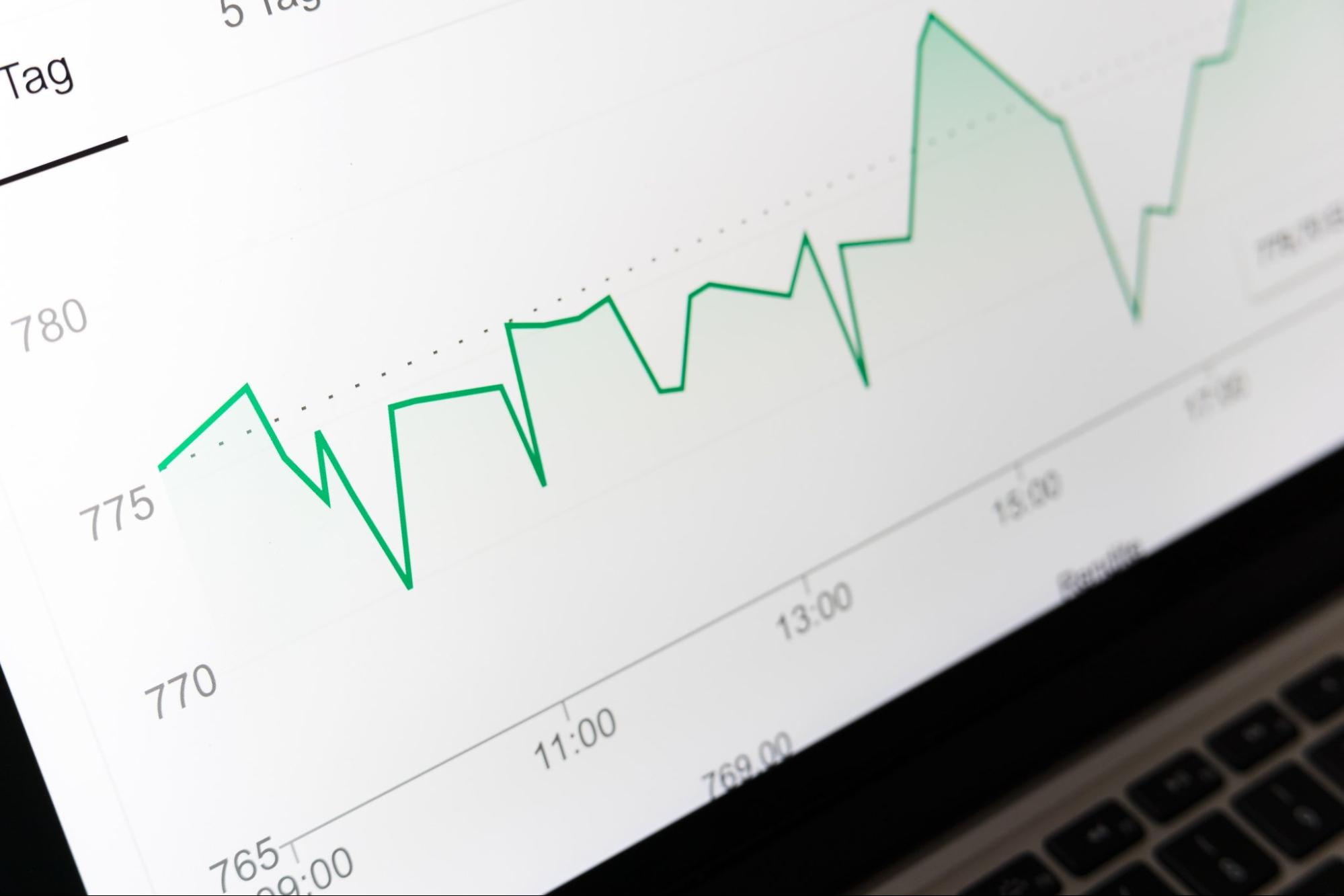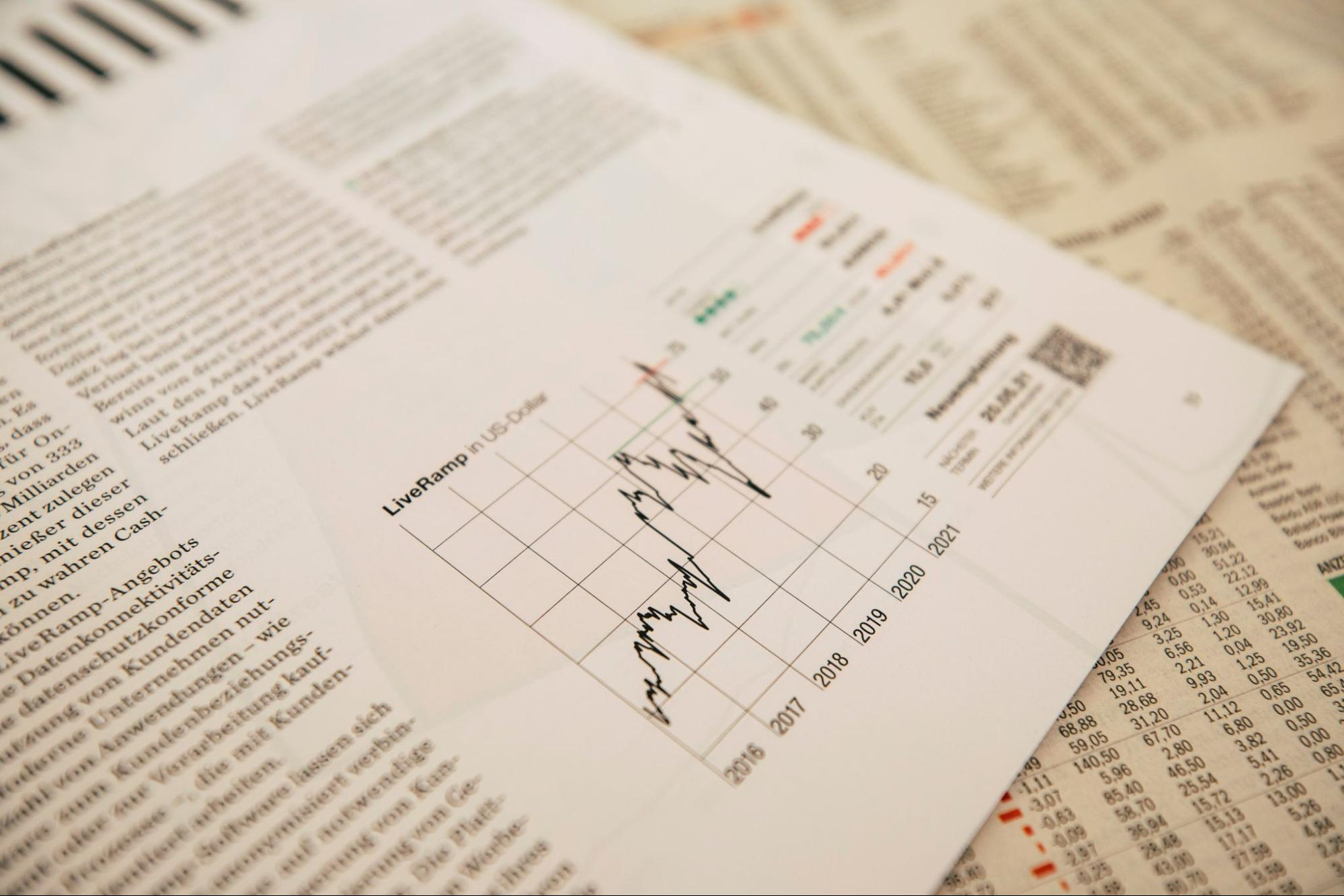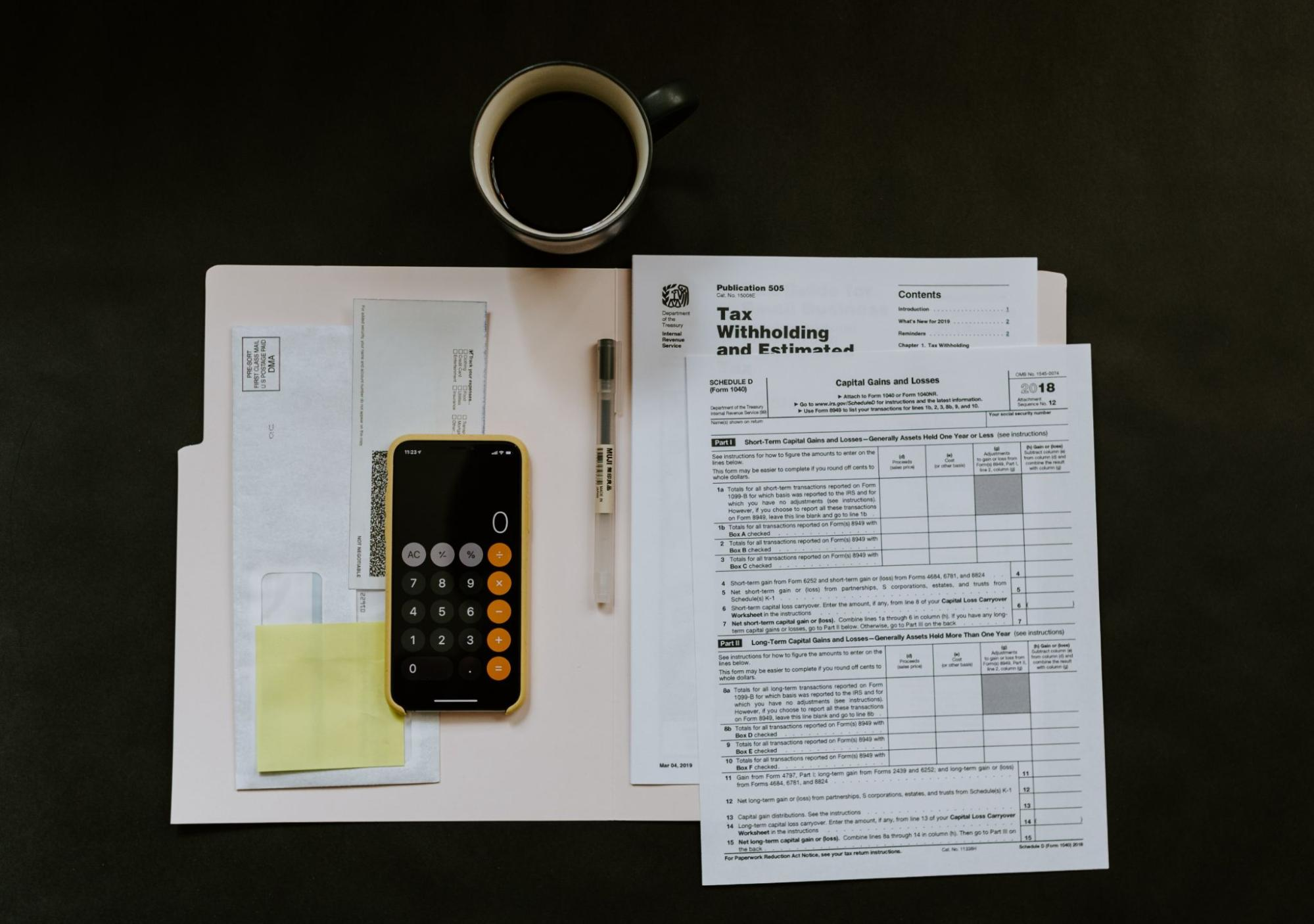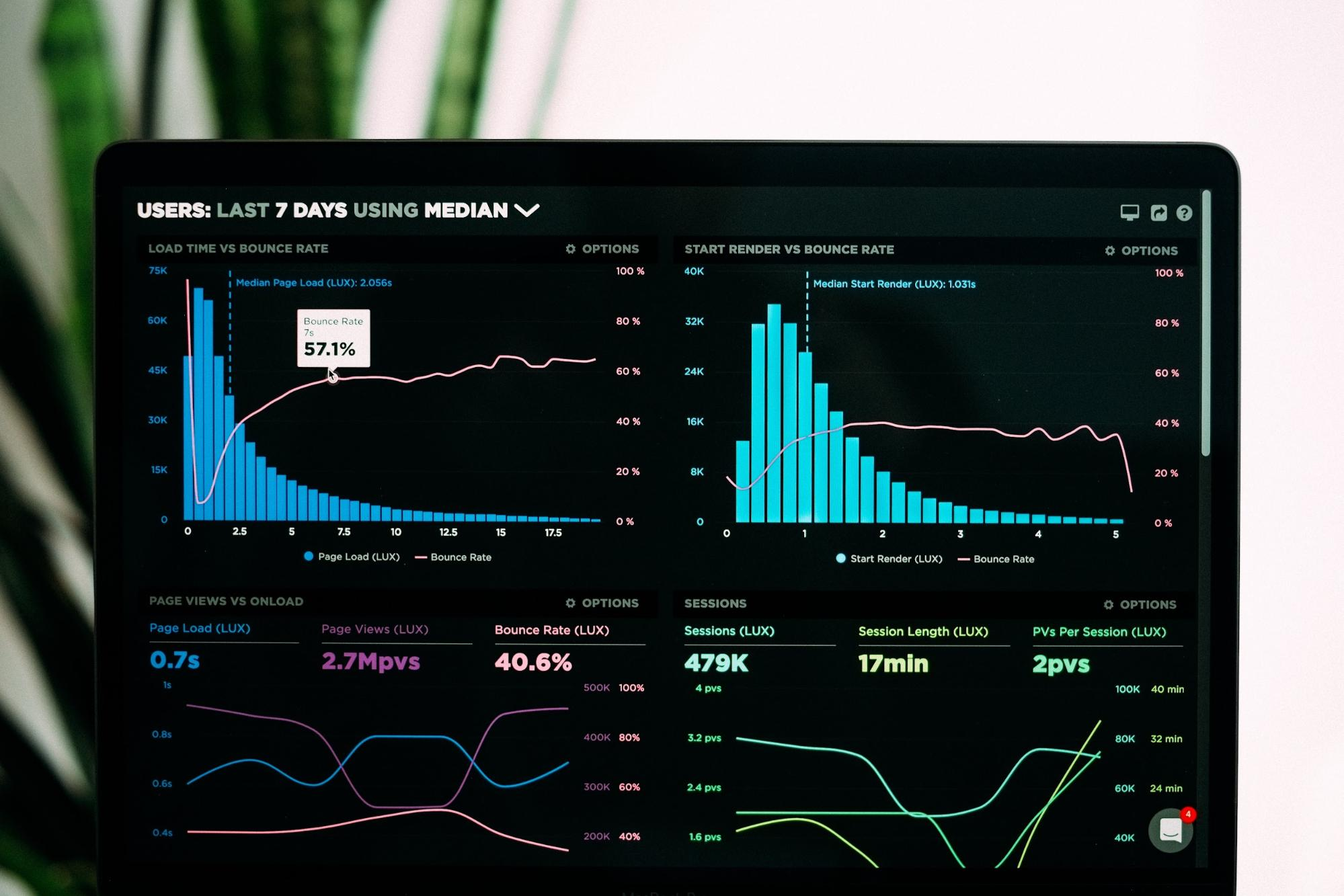# Accounting Profit vs Economic Profit

The differences two most commonly used forms of profit

To talk about the difference between economic and account profit, we ought to comprehend the notion of "profit". What is profit?Is it the sales a business makes, or is it the money left over after accounting for expenses? If it involves accounting for expenses, what are the expenses to be accounted for? Does profit mean the same thing to all stakeholders in a business? For example, are dividends deducted to arrive at a profit?

To answer these questions, we'll take you through Knight's profit theory and two additional theories complementary to the former.

At a fundamental level, we can define profit as the difference between the revenue and the costs.

Although this is the most commonly used definition, it does not describe the word profit. At least, it doesn't do enough justice to a word that carries significant depth.

There are many different types of profits in accounting, each with theoretical arguments that cater to different stakeholders and serve different needs and purposes.

## What is profit?

Economic thinking has evolved over the years, and with it, its view of profits and value. An important "revolution" occurred during the nineteenth century, known as the Marginalist Revolution.This revolution wasn't a revolution in terms of politics but a change in thought among the economists. The marginalists brought new tools to economics; marginal analysis and marginal value theory.

The marginal value of a good or service is an interesting theory widely applied today but isn't the topic of interest here.

Using marginal analysis, economists can comprehend changes with the help of derivatives (calculus).

Derivatives are nothing but a way to measure the rate of change. As a result, economists can account for dynamic factors in their models and no longer have to be stuck with static analysis.

But why is the static analysis not useful? Professor J. B Clark answers this question by pointing out the major barriers to using static assumptions in modeling, which are as follows:

1. The population is increasing.
2. Capital is changing.
3. The productive process is changing.
4. The market selects the most productive shops.
5. Consumers want to consume more and more.

These 5 simple factors change how we look at a profit in the real world. We can't deny that profit is the return on capital in its basic form.

However, when we add the dynamic factors to our analysis, profit can take on forms such as marginal profit (which is more important than vanilla profit when it comes to a business deciding on what and how much to produce).

One of the main factors that affect profits is costs. In this next section, we look at the various costs and the accounting and economics look at it while calculating profits.

## Costs in economics vs. costs in accounting

But why are they different? First, the math behind profit is the same, i.e., revenue minus costs. Hence, for profit to be different, either the way in which revenue is calculated or cost is considered must be different.The revenue is always the same, i.e., the number of goods or services sold multiplied by its price.

Hence, it isn't the reason for the difference between both profits.

If revenue isn't the reason for the difference, it only follows that the difference between accounting and economic profits is due to the differences in costs.

But how can "cost" mean a different thing in two sciences?

Quick spoiler; in accounting, we're dealing with the enterprise, its operation, and its financial health (s your company paying itself and remaining money?), while in economics, we're most concerned about the problem of profit maximization (what is the most profitable thing you could do?)

We are more accustomed to accounting profit in our day-to-day. Thus, being more specific, the problem here is to learn why economists deal with profits in the way they do.

In the sections to follow, we answer this and many other questions you may have on the topic. But, first, we will start with the accounting view of profit and then link it to the economic view.

But don't worry if you want it the other way round. The last two sections will have examples to help us understand their connection.

### Expenses in accounting

When analyzing an income statement, we see many different kinds of profits. This is because accounting deals with different costs and expenses that mean different things to various stakeholders.For example, tax expense affects the equity shareholders but does not affect the returns to the debt holders (as interest is a tax-deductible expense).

Further, expenses can be divided into fixed and variable. Below are brief definitions to help you understand them better:

• Variable Costs are costs that change based on the level of production. Think, "if I don't produce anything in a month, what I won't pay".
• Fixed costs are costs that don't change with production and have to be paid regardless of whether you actually produce or sell anything.

After understanding these concepts, it will be easier to understand accounting profits.

### Expenses in Economics

In Economics, we don't have this worry with the classification of costs, expenses, fixes, variables, etc. It's much easier (for now). If you look at any chapters on microeconomic theory on production, you'll see that it's all related to costs.A simplified production function has only two inputs, capital (K) and labor (L), and their costs are n and w, respectively. Using these, the total cost (C) is given by the following formula:

C = wL + rK

• Cost of labor (w): Accountants would break this into salaries, commissions, benefits, etc.
• Economists don't care about the department or the kind of payment. It's all labor's cost.
• Cost of capital (n): Unlike accountants, economists do not consider the amount paid to acquire machinery or its depreciation as costs. Instead, they consider cost as the amount that could be received by renting out the capital to a third party.

Economists don't ignore the cost of buying the machinery. Instead, they consider it a sunk cost, i.e., there is no way to recover it, and thus, it is irrelevant to the production decisions.

Economic costs deal with a concept called opportunity costs instead. Let's understand opportunity costs using an example.

Suppose you had a job that pays you US 200,000 a year, but now you want to start a business. You estimate that your business will be able to generate a net profit of \$125,000 annually. From an accounting sense, you generate a profit.

However, does it make sense for you to quit your job and start a business? Of course not.

In this case, it is the \$200,000 salary you receive from your job, which is known as the opportunity cost.

Keeping this in mind, setting up a business sets you back by \$75,000 annually (\$200,000 - \$125,000), and hence, is not a good idea.

Opportunity cost accounts for the limited amount of resources that we can use.

Limited resources mean that we have to make decisions based on certain trade-offs which come from not being able to use the said resources for an alternative purpose.

Our choices have a hidden cost, not necessarily monetary or time-based, and that is the cost of making that particular choice.

This cost is what is known as opportunity cost. It accounts for the alternatives given up on making a particular choice.

While making business decisions, opportunity cost is one of the most important things to consider.

Why would you open a company if you could generate better returns on the stock market?

This isn't restricted to the business world; choices and limited resources are essential in our lives.

For example, a student will find good use of opportunity costs. The time they spend studying mathematics could've been used to study philosophy, which may be a particularly time-consuming study area.

## Calculating accounting profit

Imagine running your own business, and it's the end of the quarter, and you have to calculate the quarterly profit. Where should you start?All income statements start with revenue, calculated as quantity sold (q) times the price (p).

Revenue = q * p

You don't have production costs if you're a reseller, but is it free what you buy to sell? To find the gross profit (GP) given the cost of goods sold (COGS), we can use the following formula:

GE = NR - COGS

If you have a negative GP, the revenue you generate does not cover the good's costs. If this is the case, it's usually a good idea to rethink your business model.

On the other hand, if it is equal to 0, you're selling your products at cost, which is usually the case when you've just set up and are looking to break into the market.

Next, we deduct the operating expenses (Opex) that arise due to running the business. These are expenses such as employee wages, utility bills, etc., that do not directly help produce goods and services. This leads to your operating profits.

The following formula can summarize this calculation:

Operating profits = GE - Opex

Adding non-operating revenue (if any) to operating profits leads us to EBIT.

EBIT = Operating profits + Non-operating income

Finally, we deduct taxes (T) and interest (I). As Benjamin Franklin once said, "nothing is certain but death and taxes". Deducing them leads us to Net Profits (NP).

NP = EBIT - I - T

Net Profit is known as accounting profits or book profits.

Let's now look at an example to understand the calculations better. We use parenthesis in accounting to denote a negative figure and will do so in the following example.

To keep it simple, let's use only one product whose details are as follows:

• In the first quarter of the year, you've sold US\$ 50,000.00;
• The total cost of goods sold was US\$15,000.00.;
• You paid US\$ 5,000.00 in admin salaries and US\$ 10,000.00 to the other employees;
• The rent was US\$3,000.00;
• Electricity, water, and internet were US\$ 1,000.00;
• Your assets depreciated US\$ 500.00;
• You are required to pay 20% in income taxes.
• What are your earnings in the quarter?

Revenue = US\$50,000.00

(-) Production Costs = (US\$15,000.00)

Gross Profit = US\$35,000.00

(-) Wages = (US\$15,000.00)

(-) Rent = (US\$3,000.00)

(-) Electrical energy, water, and internet = (US\$1,000.00)

(-) Depreciation = (US\$500.00)

Operational Earnings = US\$15,500.00

Non-operational Gains = US\$ 0

(-) Non-operation Expenses = US\$ 0

EBIT = US\$15,500.00

(-) Income Tax = US\$ 3,100.00

Net Profit = US\$ 12,400

## Economic Profit: How to calculate?

We will use the same example from the previous section to ensure comparability. However, we will be calculating economic profit now, which requires more details.Following are the details from the previous example, along with some new ones that will require to calculate economic profit:

• In the first quarter of the year, you've sold US\$ 50,000.00;
• The total cost of goods sold was US\$15,000.00.;
• You paid US\$ 5,000.00 in admin salaries and US\$ 10,000.00 to the other employees;
• The rent was US\$3,000.00;
• Electricity, water, and internet were US\$ 1,000.00;
• Your assets depreciated US\$ 500.00;
• You are required to pay 20% in income tax.
• NEW: If you rented your machines could've made a total of US\$ 2,000.00. However, you wouldn't be able to use them.
• NEW: If you were working a job, you could have easily made US\$ 5,000.00, but that would mean not being able to run the business.

To avoid using a lot of theory, we purposely ignore concepts like production inputs, which should help us understand the concept better.

Let's now find out what the economic profits are.

In a straightforward generalization, the economic profit can be calculated as:

Economic Profit = Accounting Profit - Opportunity Cost

Let's see this in practice, using the details provided in the example:

Revenue = US\$50,000.00

(-) Production Costs = (US\$15,000.00)

Gross Profit = US\$35,000.00

(-) Wages = (US\$15,000.00)

(-) Rent = (US\$3,000.00)

(-) Electrical energy, water, and internet = (US\$1,000.00)

(-) Depreciation = (US\$500.00)

Operational Earnings = US\$15,500.00

Non-operational Gains = US\$ 0

(-) Non-operation Expenses = US\$ 0

EBIT = US\$15,500.00

(-) Income Tax = US\$ 3,100.00

Accounting Profit = US\$ 12,400

(-) Opportunity cost

Rent from machinery forgone= (US\$ 2,000.00)

Potential wage forgone = (US\$ 5,000.00)

Economic Profit = US\$ 5,400.00

Accounting profit helps us understand if the business is profitable.

However, economic profit goes one step ahead and helps us understand whether it is better to run a business or work elsewhere. It all boils down to your needs, as each has its unique use cases.### Everything You Need To Build Your Accounting Skills

To Help You Thrive in the Most Flexible Job in the World.

Researched and authored by Luiz Eduardo de Lima LinkedIn